Next: Lagrange's Equation Up: Lagrangian Dynamics Previous: Generalized Coordinates

Generalized Forces

The work done on the dynamical system when its Cartesian coordinates change by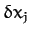is simply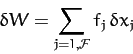(594)

Here, the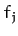are the Cartesian components of the forces acting on the various particles making up the system. Thus,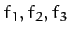are the components of the force acting on the first particle,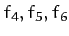the components of the force acting on the second particle, etc. Using Equation (593), we can also write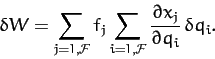(595)

The above expression can be rearranged to give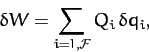(596)

where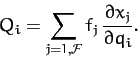(597)

Here, the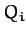are termed generalized forces. Note that a generalized force does not necessarily have the dimensions of force. However, the product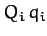must have the dimensions of work. Thus, if a particular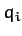is a Cartesian coordinate then the associatedis a force. Conversely, if a particularis an angle then the associatedis a torque.

Suppose that the dynamical system in question is conservative. It follows that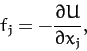(598)

for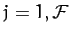, where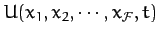is the system's potential energy. Hence, according to Equation (597),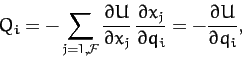(599)

for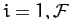.Next: Lagrange's Equation Up: Lagrangian Dynamics Previous: Generalized Coordinates
Richard Fitzpatrick 2011-03-31